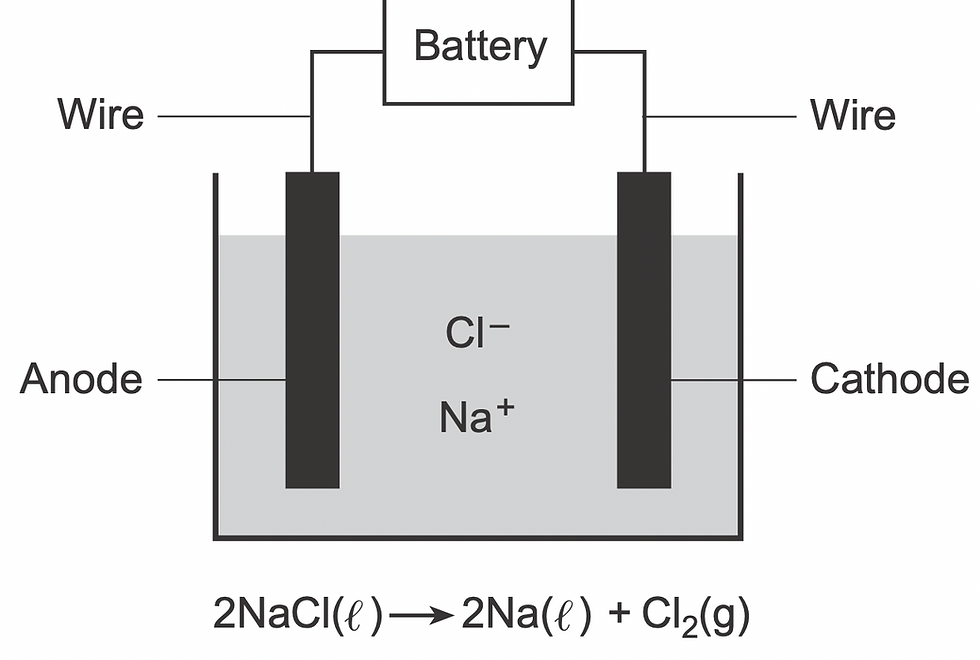top of page
Search

# The diagram and equation below represent an electrochemical cell.Which process is represented by

June 2023 NY Regents Chemistry Exam

46 The diagram and equation below represent an electrochemical cell.Which process is represented by this diagram?

(1) chromatography

(2) distillation (3) electrolysis (4) polymerization

Solution: The picture shows an electrochemical cell. There are two types of electrochemical cells: voltaic and electrolytic. Electrolytic cell uses energy to run a chemical reaction in a process called electrolysis.

Prepare for the Chemistry Regents Exam: HERE